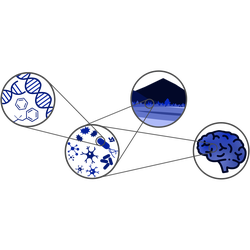# Statistical Mechanics of Complex Systems

Here you can find the course page on the university database.

Please send the exercises to suweis@pd.infn.it with object “Exercise Ing 2018”.

## Some notes on stochastic processes

Here you can find notes on stochastic processes. Here you can find notes on persistence times of a birth-death process.

## Introduction to Monte Carlo methods

• Generation of sequences of uniformly distributed (pseudo)random numbers, using different simple congruent methods, illustrating their performance. Source code.
• Generation of exponentially distributed random numbers, using the inverse method. Source code.
• Generation of Gaussian random numbers, using the Box-Muller method. Source code.
• Exercises.

## Maxwell-Boltzmann distribution for ideal gases

• Calculation of the pressure from microscopic collisions with the wall (Ex. 3.4 Sethna). We run a simulation and compute the histogram of collisions in the right wall during an interval of time, as a function of the moment carried by the particles. Source code (need to be improved!).

## Introduction to Complex Networks

• Some slides.
• Simulation: how to generate random Erdős–Rényi and small world networks (Ex. 3.7 Sethna).
• HOMEWORK (to submit by Thursday 12 April): Analyze the Marvel-Heros Social Network! If the network is too large, and you have problem in analyzing it, you can instead study the following brain network (see Exercise 2 in the homework); it is a .dat file describing the weighted adjacency matrix, so it is very easy to upload and start to work on it.

## Fractals

• Summary of the Mathematica program discussed in class (.cdf can be read also without having Mathematica: you can download CDF viewer for free in the Wolfram website) CDF Mathematica Notebook.
• HOMEWORK: exercise on fractals.

## Monte Carlo simulation of equilibrium processes

• Summary and Exercise. Deadline: 1 June 2018.
• Simulation: Metropolis algorithm for the Ising model in a 2D-lattice. Source code.
• Simulation: Cluster algorithm for the Ising model in a 2D-lattice. Source code.
• Notes and Simulation: Fractal in the Ising Model. Source code.

Bibliography: W. Krauth, Cluster Monte Carlo algorithms.

## Stochastic Interacting Particle Models and applications

• Review on the application of the Voter Model in Ecology.
• Voter Model code from Wolfram Demonstration Project.
• Notes and code on stochastic reactions formalism and Gillespie algorithm.
• Notes on how to solve diffusion Equation for the voter model.

Bibliography: D. Gillespie, Stochastic Simulation of Chemical Kinetics, Annual Review of Physical Chemistry.

## Examples of exams of previous years